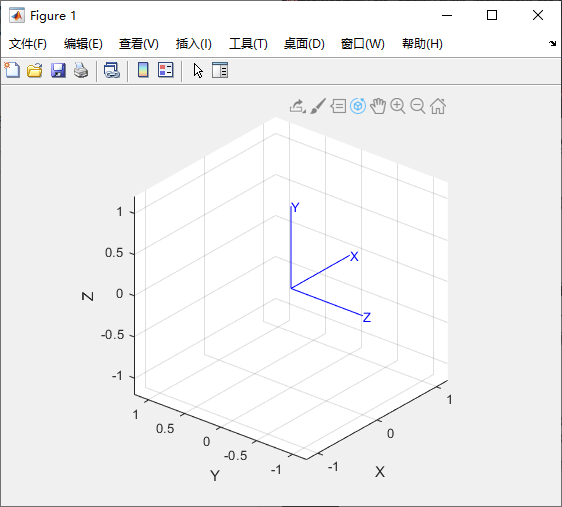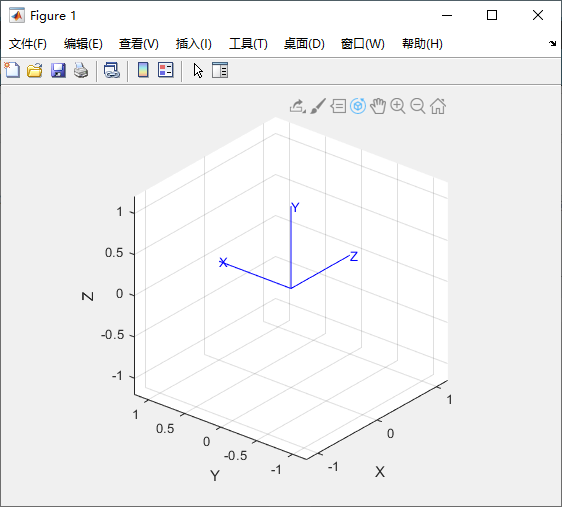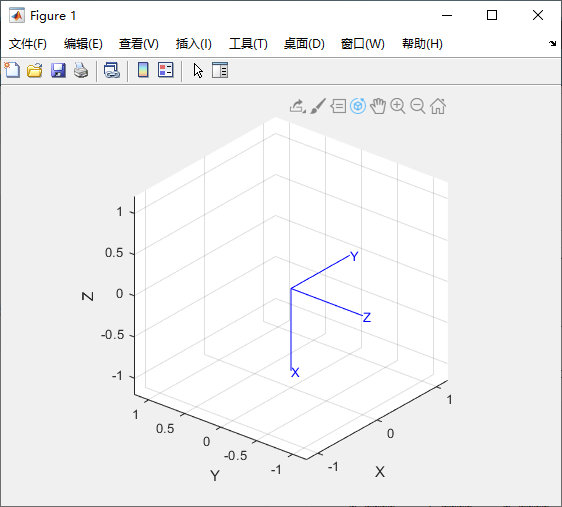。它具有前文提到的标准正交矩阵的特性，如 RT= R−1以及 det(R) = 1>> R = rotx(pi/2)
R =
1.0000         0         0
0    0.0000   -1.0000
0    1.0000    0.0000


>> trplot(R)>> tranimate(R)


R =
1.0000         0         0
0    0.0000   -1.0000
0    1.0000    0.0000


>> R = rotx(pi/2) * roty(pi/2)
R =
0.0000         0    1.0000
1.0000    0.0000   -0.0000
-0.0000    1.0000    0.0000
>> trplot(R)>> R = roty(pi/2) * rotx(pi/2)
R =
0.0000    1.0000    0.0000
0    0.0000   -1.0000
-1.0000    0.0000    0.0000
>> trplot(R)